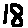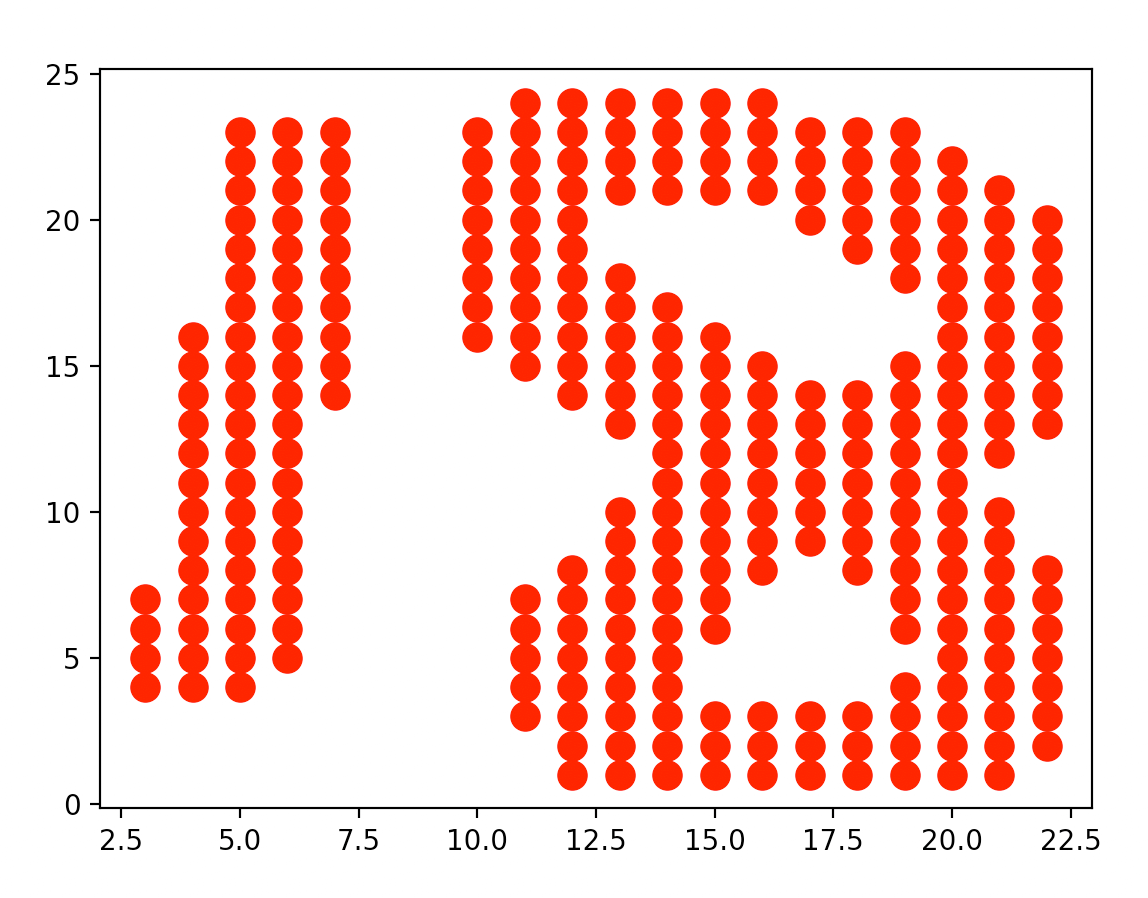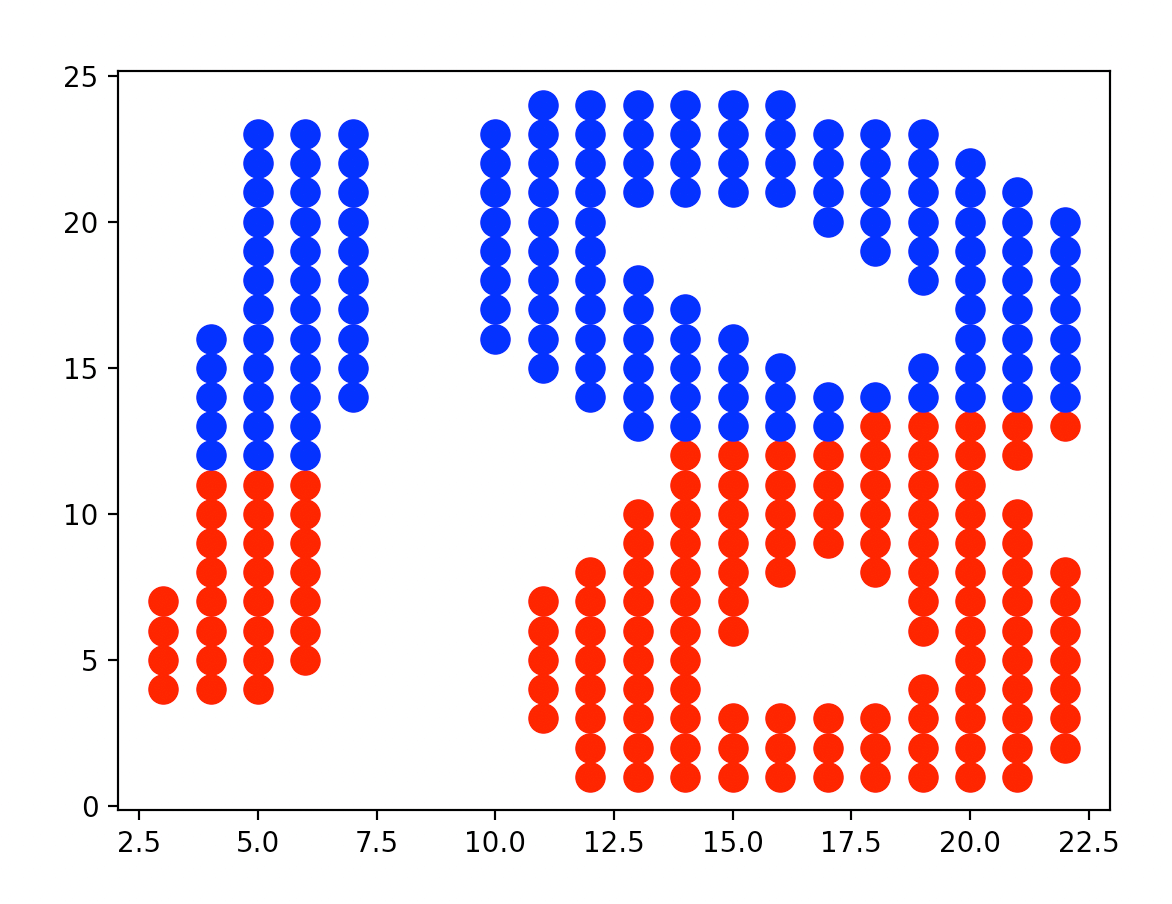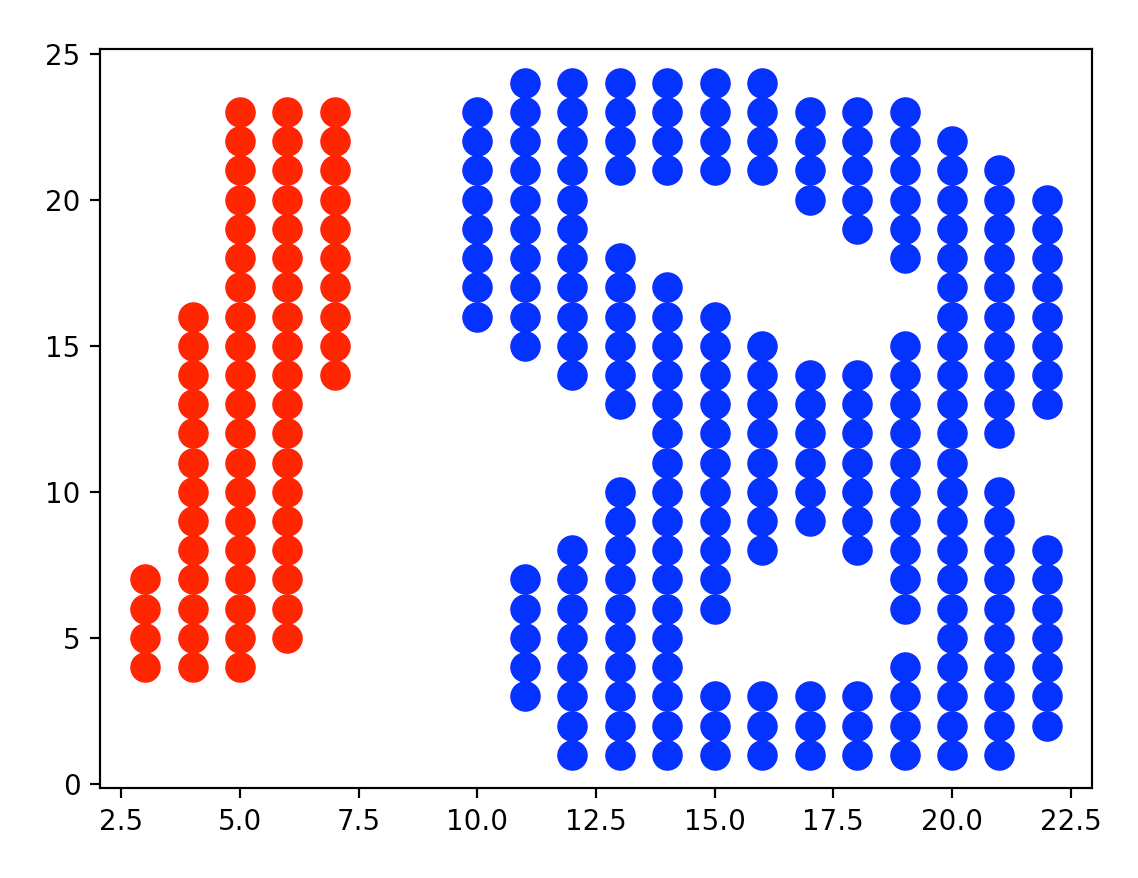# 【Python基础】第三十九课：使用Python实现DBSCAN聚类

## sklearn.cluster.DBSCAN，PIL模块，sklearn.preprocessing.binarize，np.where，np.column_stack

Posted by x-jeff on August 12, 2022

# 1.DBSCAN算法

K-means比较：

• 优点：
• K-means方法相比，DBSCAN不需要事先知道K。
• K-means方法相比，DBSCAN可以找到任意形状。
• DBSCAN能够识别出噪声点。
• DBSCAN对于数据库中样本的顺序不敏感。
• 缺点：
• DBSCAN不适合反映高维度资料。
• DBSCAN不适合反映已变化数据的密度。

# 2.sklearn.cluster.DBSCAN

1
2
3
4
5
6
7
8
9
10
11
def __init__(
self,
eps=0.5,
min_samples=5,
metric='euclidean',
metric_params=None,
algorithm='auto',
leaf_size=30,
p=None,
n_jobs=1
)


1. eps：即DBSCAN算法中的邻域参数中的$\epsilon$。
2. min_samples：即DBSCAN算法中的邻域参数中的MinPts。
3. metric：距离计算方式，默认为欧式距离。可以使用的距离度量参数有euclidean，manhattan，chebyshev，minkowski，wminkowski，seuclidean，mahalanobis。
4. metric_params：距离计算的其他关键参数，默认为None。
5. algorithm：有auto，ball_tree，kd_tree，brute四种选择。DBSCAN算法中会用到最近邻算法，algorithm参数为最近邻算法的求解方式。
6. leaf_size：当algorithm为ball_tree或kd_tree时，该参数用于指定停止建子树的叶子节点数量的阈值。
7. p：当metric使用闵可夫斯基距离时，p即为距离公式中的$p$。
8. n_jobs：CPU并行数，值为-1时使用所有CPU运算。

# 3.实战应用👉将图像读取成numpy array：

1
2
3
4
5
6
import numpy as np
from PIL import Image

img = Image.open("handwriting.png")
img2 = img.rotate(-90).convert("L")
imgarr = np.array(img2)


PIL模块定义了9种图像模式：

1. “1”：1位像素，非黑即白，即二值图像。
2. “L”：8位像素，灰度图像。
• 从模式”RGB”转换为模式”L”是按照此公式转换的：$L = R * 299 / 1000 + G * 587/1000 + B * 114 /1000$。
3. “P”：8位像素，彩色图像。其对应的彩色值是按照调色板查询出来的。
4. “RGBA”：32位彩色图像。其中24位表示R、G、B三个通道，另外8位表示alpha通道，即透明通道。当模式”RGB”转为模式”RGBA”时，alpha通道全部设置为255，即完全不透明。
5. “CMYK”：32位彩色图像。它是印刷时采用的四分色模式：
• C = Cyan：青色，天蓝色，湛蓝。
• M = Magenta：品红色，洋红色。
• Y = Yellow：黄色。
• K = Key plate (blacK)：黑色。
• 将模式”RGB”转为模式”CMYK”的公式如下：
• $C=255-R$
• $M=255-G$
• $Y=255-B$
• $K=0$
6. “YCbCr”：24位彩色图像。
• Y指亮度分量。
• Cb指蓝色色度分量。
• Cr指红色色度分量。
• 模式”RGB”转为模式”YCbCr”的公式如下：
• $Y= 0.257*R+0.504*G+0.098*B+16$
• $Cb = -0.148*R-0.291*G+0.439*B+128$
• $Cr = 0.439*R-0.368*G-0.071*B+128$
7. “I”：32位整型灰度图像。模式”RGB”转换为模式”I”参照如下公式（和模式”L”的公式一样）：
• $I = R * 299/1000 + G * 587/1000 + B * 114/1000$
8. “F”：32位浮点型灰度图像。模式”RGB”转换为模式”F”所用的公式和模式”L”（或模式”I”）都是一样的。
9. “RGB”：$3\times 8$位彩色图像。

👉将图像归一化：

1
2
from sklearn.preprocessing import binarize
imagedata = np.where(1 - binarize(imgarr, 0) == 1)


sklearn.preprocessing.binarize(X, threshold=0.0, copy=True)参数详解：

1. X：稀疏矩阵，array。大小为[n_samples, n_features]
2. threshold：可选参数，默认为0.0。小于等于threshold的值被置为0，否则置为1。
3. copy：如果为False，则结果覆盖X。如果为True则不覆盖。

np.where有两种用法：

1. np.where(condition, x, y)：当np.where有三个参数时，第一个参数表示条件，当条件成立时，where方法返回x，当条件不成立时where返回y。例如：np.where(X >= 0.0, 1, 0)
2. np.where(condition)：当where内只有一个参数时，那个参数表示条件，当条件成立时，where返回的是每个符合condition条件元素的坐标，返回的是以tuple的形式。

👉可视化：

1
2
3
import matplotlib.pyplot as plt
plt.scatter(imagedata, imagedata, s=100, c='red', label="Cluster 1")
plt.show()👉使用KMeans聚类：

1
2
3
4
from sklearn.cluster import KMeans
X = np.column_stack([imagedata, imagedata])
kmeans = KMeans(n_clusters=2, init="k-means++", random_state=42)
y_kmeans = kmeans.fit_predict(X)


np.column_stack将两个矩阵按列合并。KMeans函数的使用方法见：KMeans函数

👉呈现KMeans聚类结果：

1
2
3
plt.scatter(X[y_kmeans == 0, 0], X[y_kmeans == 0, 1], s=100, c="red", label="Cluster 1")
plt.scatter(X[y_kmeans == 1, 0], X[y_kmeans == 1, 1], s=100, c="blue", label="Cluster 2")
plt.show()👉使用DBSCAN聚类：

1
2
3
4
5
6
7
from sklearn.cluster import DBSCAN
dbs = DBSCAN(eps=1, min_samples=3)
y_dbs = dbs.fit_predict(X)

plt.scatter(X[y_dbs == 0, 0], X[y_dbs == 0, 1], s=100, c="red", label="Cluster 1")
plt.scatter(X[y_dbs == 1, 0], X[y_dbs == 1, 1], s=100, c="blue", label="Cluster 2")
plt.show()# 5.参考资料